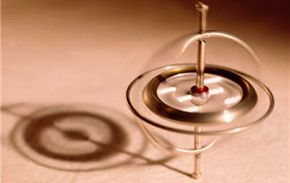# Debunking The Perpetual Motion Myth: What is zero-point energy?How much do you know about perpetual motion? iStockphoto/Thinkstock

Imagine an energy source that was infinite, clean, and completely scalable. It would solve many of the world's problems -- and sounds too good to be true. This is the promise of "zero-point energy" - the idea that the lowest possible energy of a system, its "ground state," can in fact be harnessed by a machine -- and, unfortunately, still very much in the realm of science fiction.

## An Accepted Idea

The concept of zero-point energy, as a phenomena of quantum mechanics, is in fact accepted by physicists and is not considered controversial. The most accessible example of zero-point energy is the vacuum. A vacuum is simply the ground state -- point of lowest possible energy -- of all the fields in space.

The zero-point energy of a system fluctuates naturally, giving it a wave-like nature.

On the next page, learn about an unusual application for zero-point energy

## An Unusual Application

While the concept of zero-point energy is generally accepted, the idea that this energy can be harnessed as a power source is highly controversial. The theory is that a device could capture the zero-point energy, which is commonly considered to be infinite. This idea is, at its root, in conflict with the current understanding of the zero-point, according to physics.

The problem, it turns out, is that the zero-point is a level which a thermodynamic system can never dip below. This means that if energy could be pulled from a system at its zero-point, that point would lower instead of yielding infinite energy. Thus, a "perpetual-motion machine" based on zero-point energy would be chasing a goal it could never -- thanks to quantum mechanics’ reach.